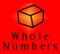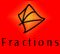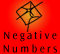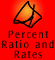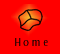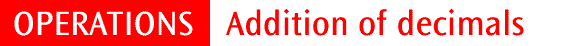| Quick Quiz |

Addition of decimals is very similar to addition of whole numbers. It is important to understand the place value of each digit, both in performing the operation and in relation to determining which zeros are important.

Example 1: Physical demonstration of 2.3 + 0.5 = 2.8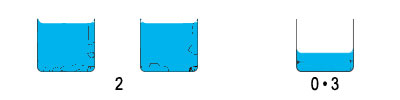2.3 is 2 litres and 0.3, or 3 tenths, of a litre.To this we add 0.5, or 5 tenths, of a litre.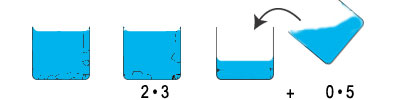We add the 3 tenths and 5 tenths, or we can say, we add 0.3 and 0.5.We can see that this is 8 tenths, or 0.8 and that, 2.3 litres plus 5 tenths of a litre is 2.8 litres.

Example 2: Using LAB to demonstrate 0.156 + 0.264 = 0.42

Example 3: Using place value columns, 2.3 + 0.06 + 1.7 = 4.06

Example 4: Expert performance of 2.746 + 1.154 = 3.9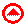1 0.7 + 0.6 2 5.792 + 4.1 3 11.805 + 0.927 4 5.76 + 0.89 + 12.046

Extra questions:

1. What is the sum of 2.84, 4.7, 35 and 8.827

2. What number is 4.93275 more than 19.067?

3. Chris runs 0.925 km then cycles 12.7 km and then walks 0.386km. How far does he go altogether?

4. Rose drank 0.875 litres of milk on Monday and on Tuesday she drank 0.26 litres more milk than she drank on Monday. How many litres of milk did she drink altogether over the two days?

5. A piece of string is 1.987 metres long and another piece of string is 2.03 metres long. What is the total length of the two pieces of string?

6. 1.839 is 12.9024 less than a certain number. What is the number?

If you would like to do some more questions, click here to go to the mixed operations quiz at the end of the division section.